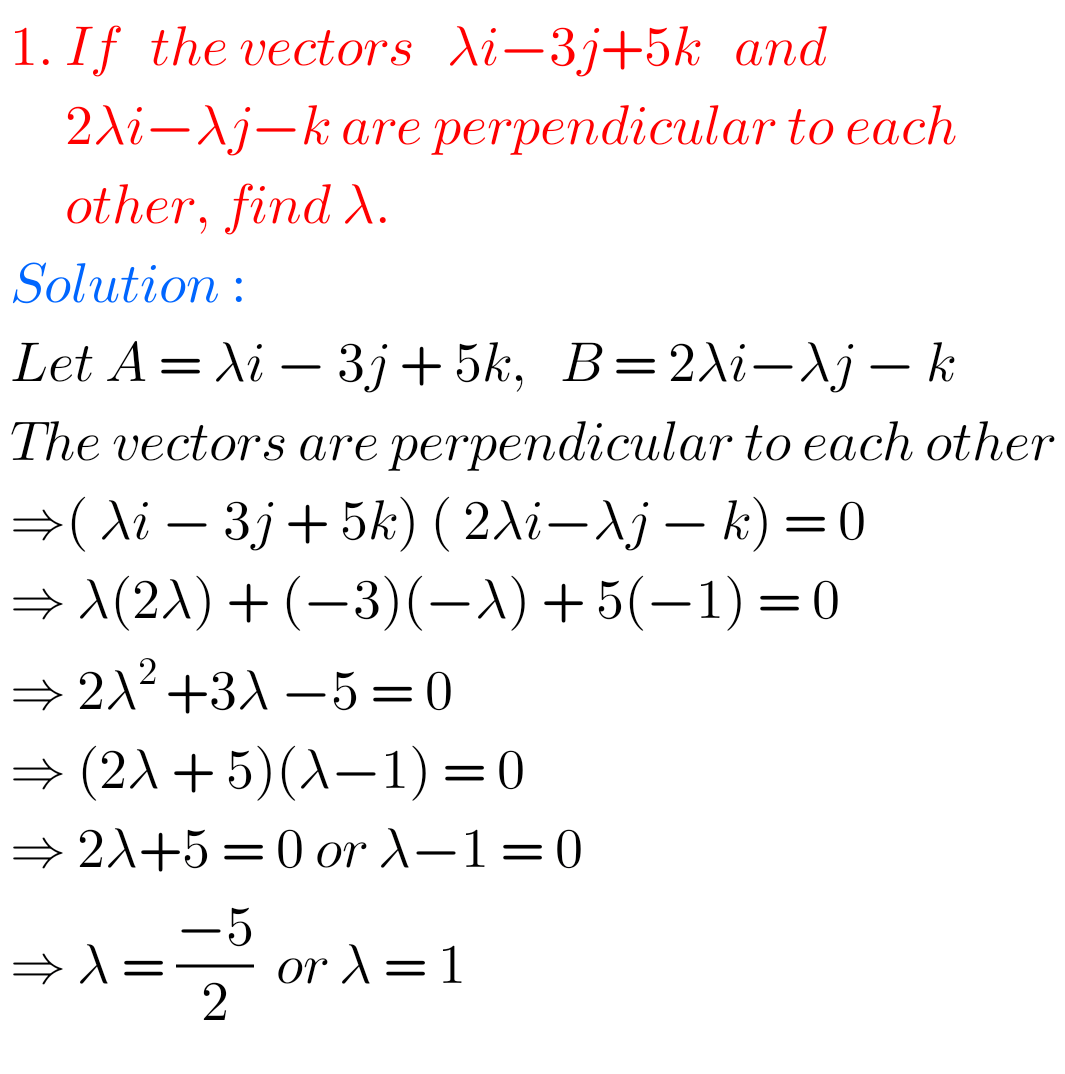# Intermediate Maths Solutions for Addition of Vectors Exercise 4(b)

Intermediate mathematics IA Exercise 1(b) Addition of Vectors textbook solutions are given.

These solutions are very easy to understand.

Then observe the example problems and solutions given in the text book. Try them.

Observe the solutions given below. Try them in your own method.

You can also see

Inter Maths 1A textbook solutions

Inter Maths 1B textbook solutions

Inter Maths IIA textbook solutions

Inter Maths IIB textbook solutions

SSC Maths text book Solutions class 10

Exercise 4(a)

Exercise 4(b)

M

## Inter Maths solutions for Exercise 4(b) Addition of Vectors

Addition of Vectors – Exercise 4(b)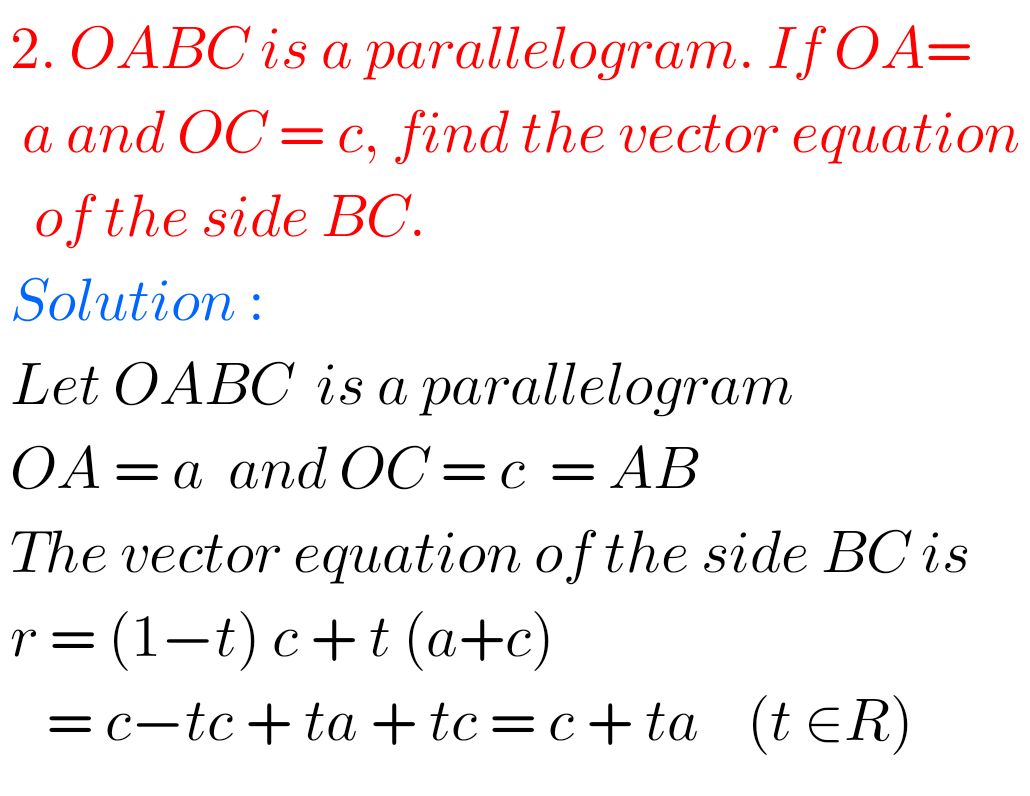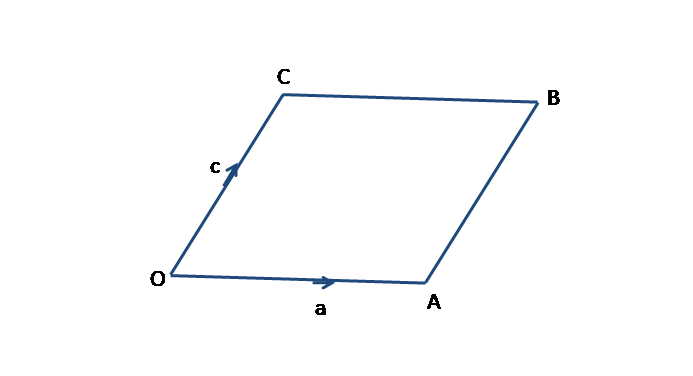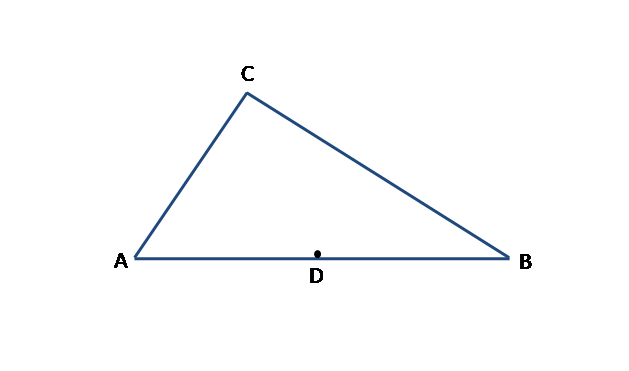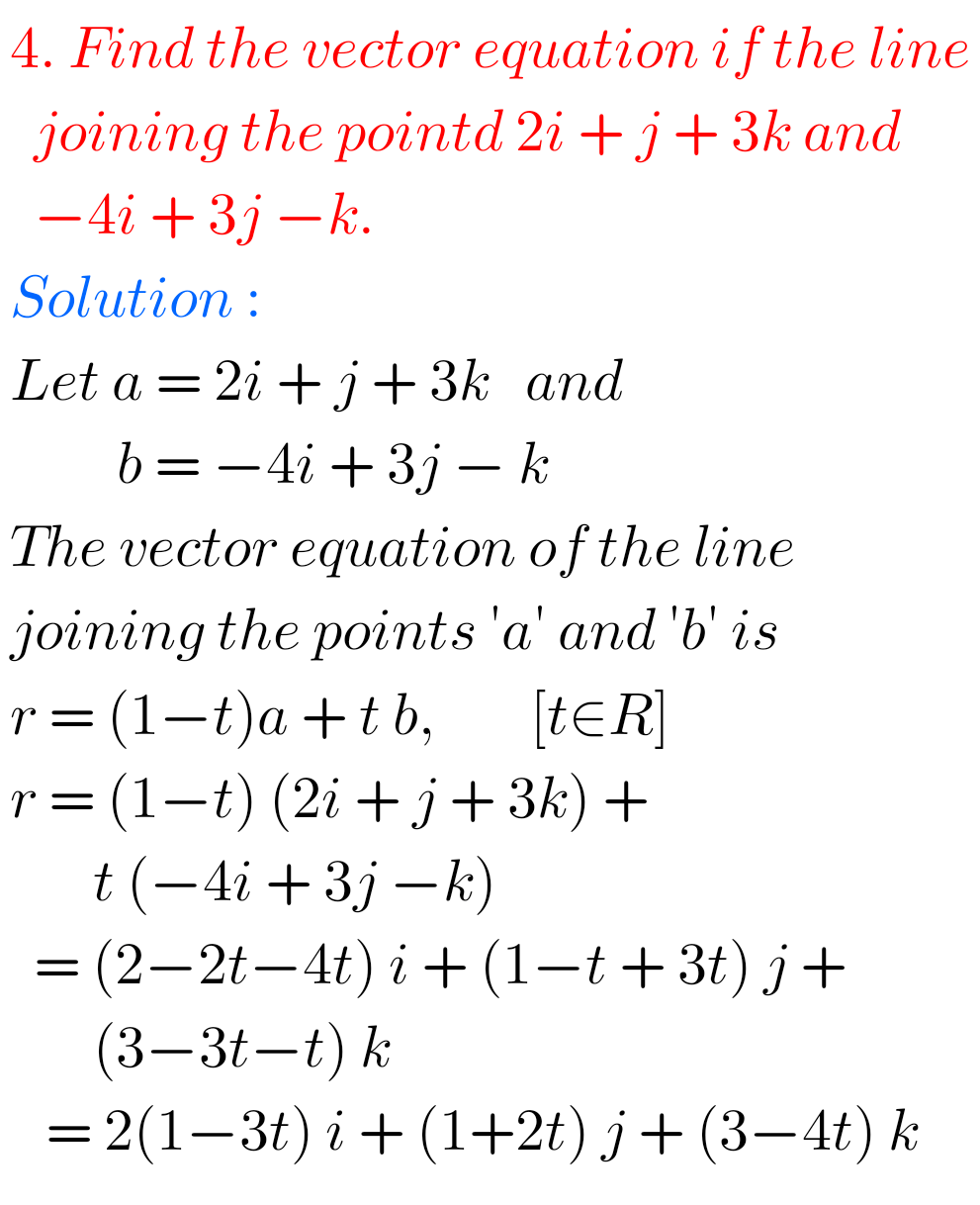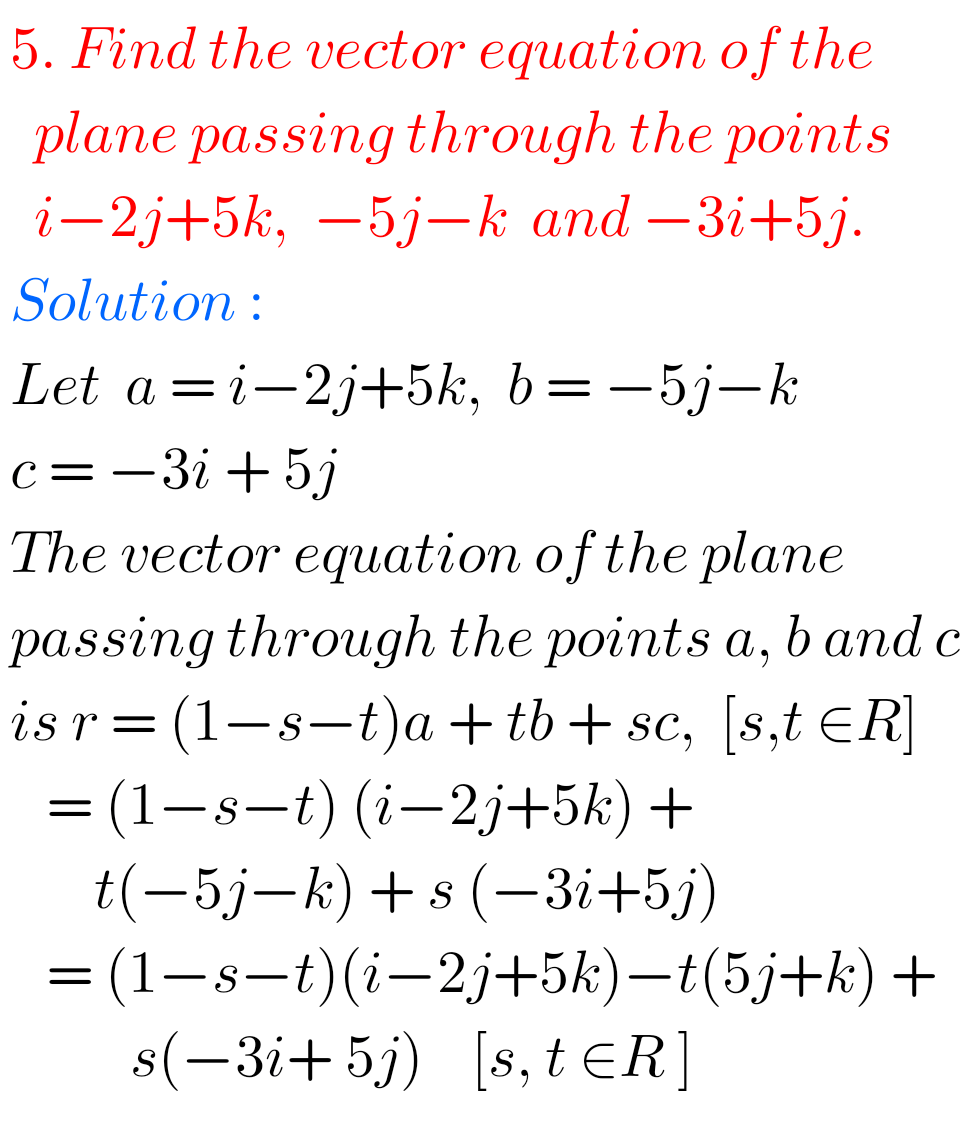## Maths Solutions for Addition of Vectors Exercise 4(b)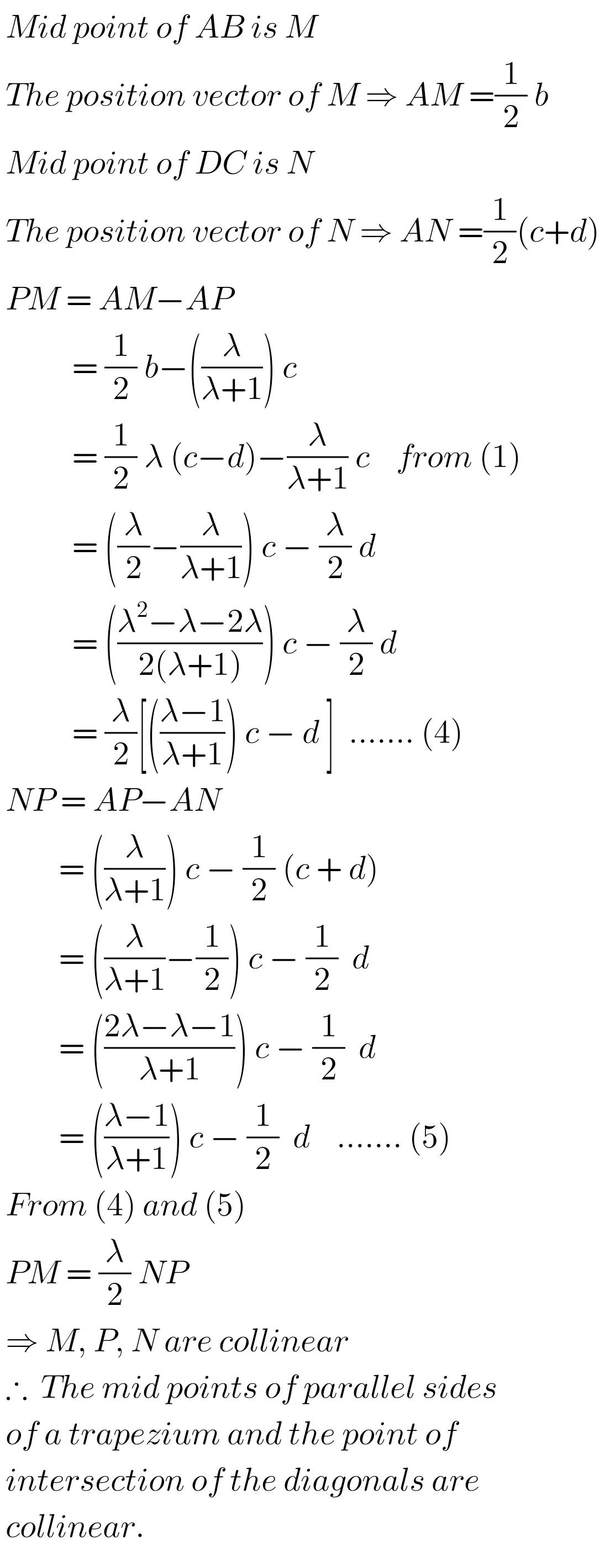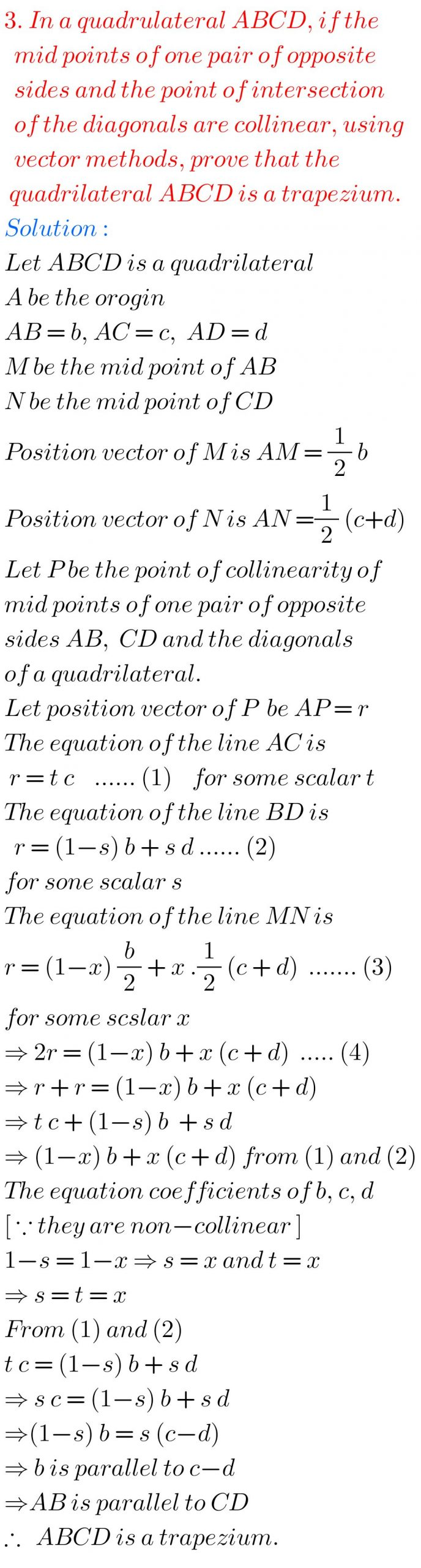## Addition of Vectors Exercise 4(b) Solutions Inter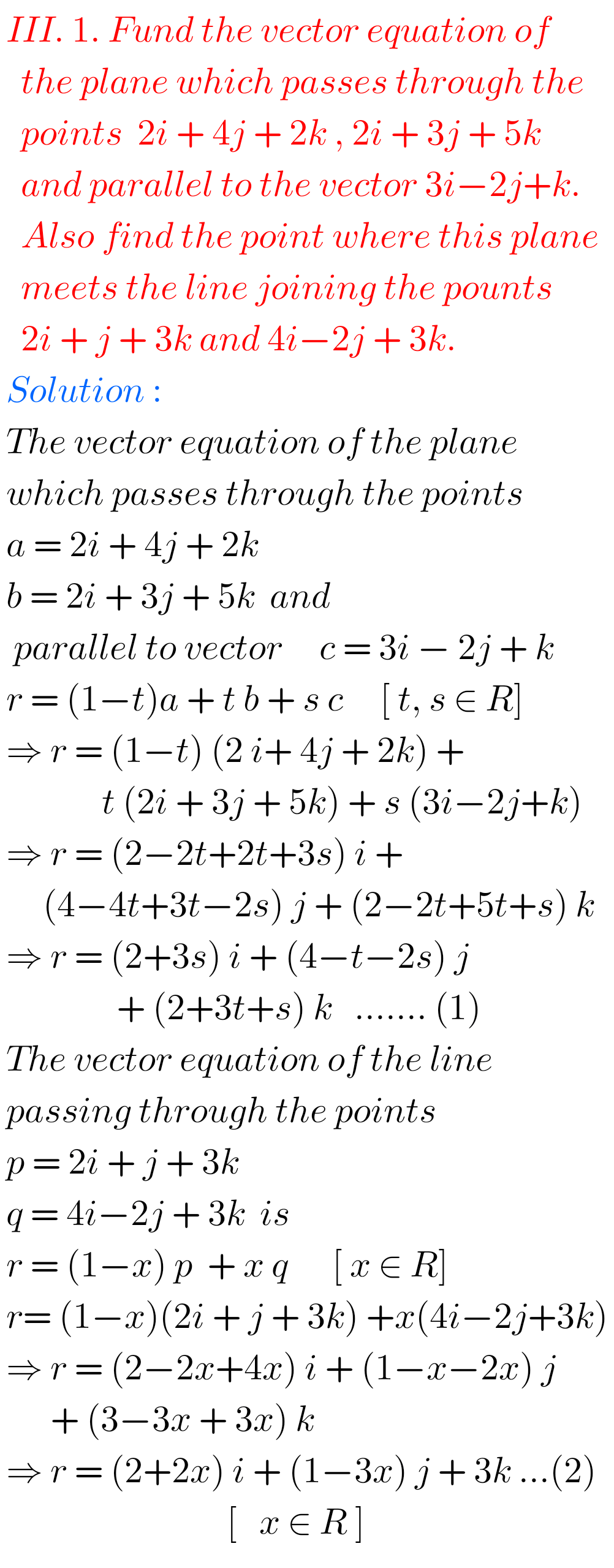Note : Observe the solutions and try them in your own method.

Some more